### Home > MC2 > Chapter 5 > Lesson 5.1.1 > Problem5-10

5-10.
1. The trapezoids below are similar shapes. Homework Help ✎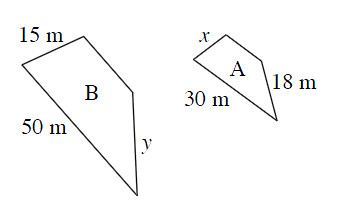1. What is the scale factor between shapes A and shape B?

2. Find the lengths of the missing sides.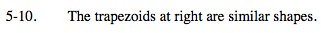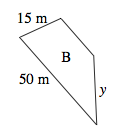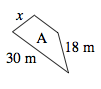Refer to problem 4-98 for help with scale factors between two shapes.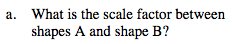What is the ratio between the pair of sides 50m and 30m?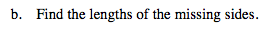Use this ratio with each other pair of sides with one given length to solve for the variable.

x = 9m

If x is 9m, what would y be? Make sure to know how to get this answer.